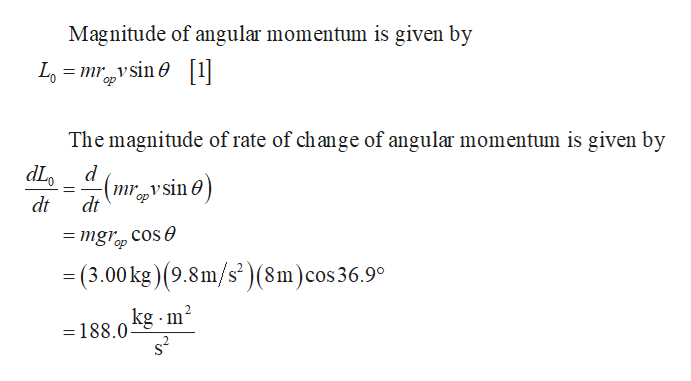A 3.00 kg rock has a horizontal velocity of magnitude 12.0 m/s when it is at point P in the figure (Figure 1).If the only force acting on the rock is its weight, what is the magnitude of the rate of change of its angular momentum at this instant?Express your answer in kilogram-meters squared per second squared.

Question

A 3.00 kg rock has a horizontal velocity of magnitude 12.0 m/s when it is at point P in the figure (Figure 1).

If the only force acting on the rock is its weight, what is the magnitude of the rate of change of its angular momentum at this instant?
Express your answer in kilogram-meters squared per second squared.
Step 1

Write the expression for magnit...help_outlineImage TranscriptioncloseMagnitude of angular momentum is given by Lomsin1 The magnitude of rate of change of angular momentum is given by dL mr vsin op dt dt = mgr cose op =(3.00 kg) (9.8m/s')(8m)cos36.9° kg m2 = 188.0 s2 fullscreen

Want to see the full answer?

See Solution

Want to see this answer and more?

Our solutions are written by experts, many with advanced degrees, and available 24/7

See Solution
Tagged in

Rotational Mechanics